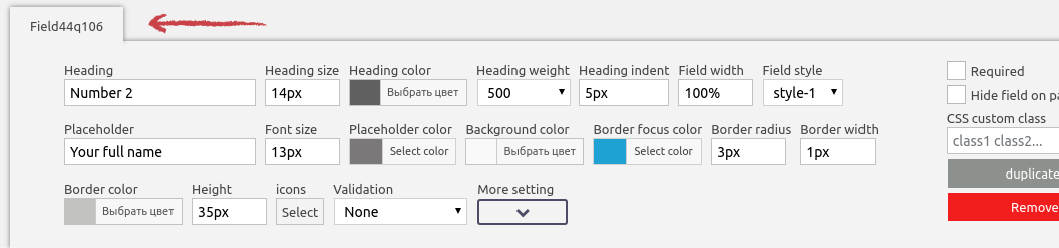## How to use math formulas

In order to create a calculator you need to add the ‘total’ field:Simple example

In the “total” field editor we see two text areas, the first contains a formula in which you specify field identifiers and mathematical operators. Basic operators are allowed in math formulas: + – / * ( )

Summarize the values ​​of the two fields in your form.Id of any field you can see in the editor: